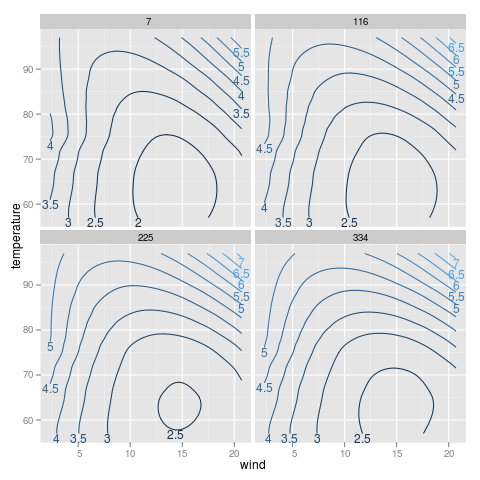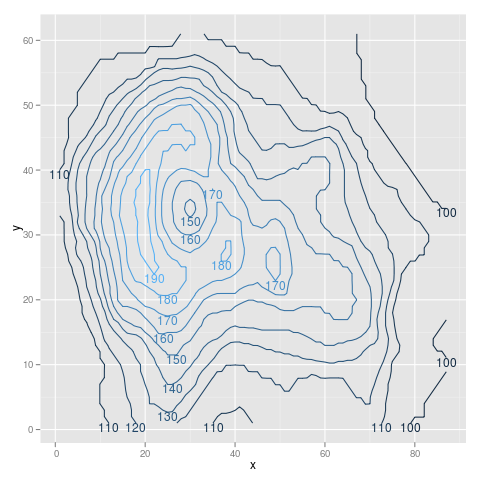# directlabels - contourplot - Positioning Method - bottom.pieces

Positioning Method for the bottom of a group of points.

```bottom.pieces <- label.pieces(which.min,1)
```environmental```## Example from help(contourplot) require(stats) require(lattice) attach(environmental) ozo.m <- loess((ozone^(1/3)) ~ wind * temperature * radiation, parametric = c("radiation", "wind"), span = 1, degree = 2) w.marginal <- seq(min(wind), max(wind), length.out = 50) t.marginal <- seq(min(temperature), max(temperature), length.out = 50) r.marginal <- seq(min(radiation), max(radiation), length.out = 4) wtr.marginal <- list(wind = w.marginal, temperature = t.marginal, radiation = r.marginal) grid <- expand.grid(wtr.marginal) grid[, "fit"] <- c(predict(ozo.m, grid)) detach(environmental) library(ggplot2) p <- ggplot(grid,aes(wind,temperature,z=fit))+ stat_contour(aes(colour=..level..))+ facet_wrap(~radiation) direct.label(p,"bottom.pieces") ```volcano```## example from help(stat_contour) library(reshape2) volcano3d <- melt(volcano) names(volcano3d) <- c("x", "y", "z") library(ggplot2) p <- ggplot(volcano3d, aes(x, y, z = z))+ stat_contour(aes(colour = ..level..)) direct.label(p,"bottom.pieces") ```
 Please contact Toby Dylan Hocking if you are using directlabels or have ideas to contribute, thanks! Documentation website generated from source code version 2021.2.24 (git revision bb6db07 Mon, 14 Jun 2021 22:38:45 +0530) using inlinedocs. validate